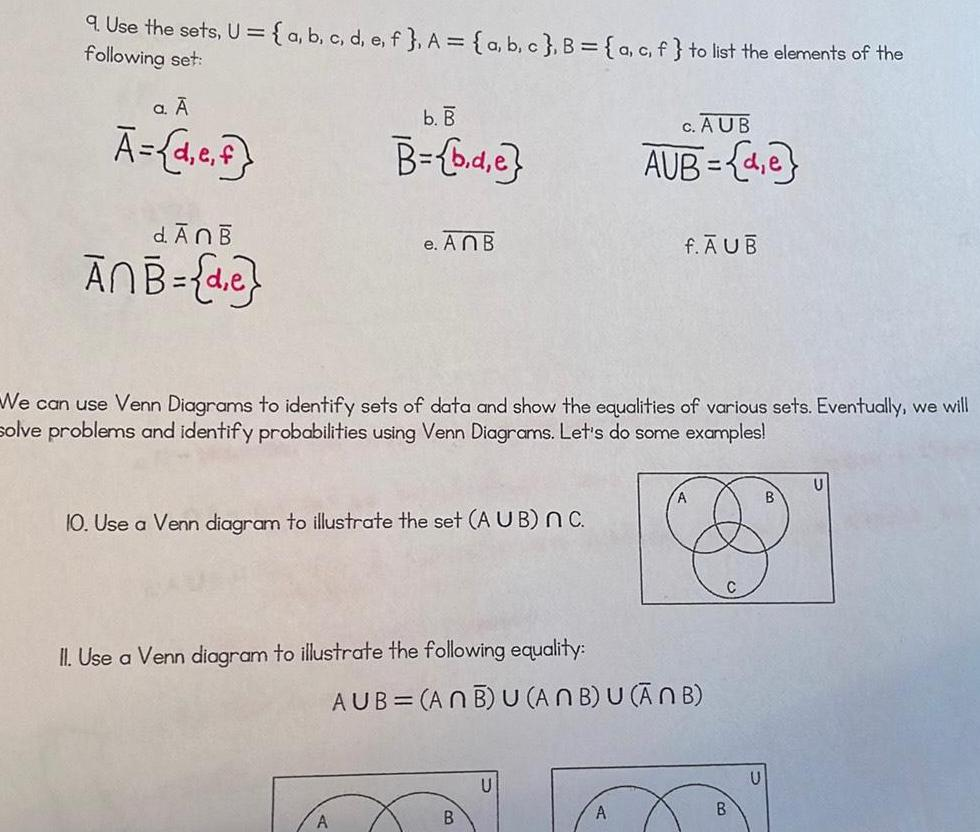Question:

# 9 Use the sets U following set a d e f a b c d e f A a b c B

Last updated: 11/15/20239 Use the sets U following set a d e f a b c d e f A a b c B a c f to list the elements of the d An B ANB de b B B b d e e An B 10 Use a Venn diagram to illustrate the set AUB nC A We can use Venn Diagrams to identify sets of data and show the equalities of various sets Eventually we will solve problems and identify probabilities using Venn Diagrams Let s do some examples II Use a Venn diagram to illustrate the following equality B C AUB AUB de U f AUB AUB ANB U ANB U ANB A A B B U